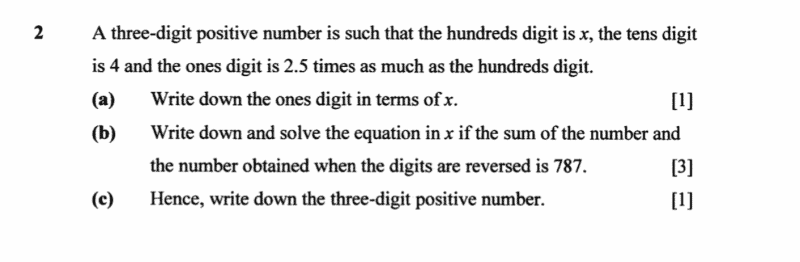# Questionthank you x

0 Replies 1 Like ✔Accepted Answer

a) The 3 digit number _ _ _
Since the hundreds place digit is X and the ones place is 2.5 times as much. The ones place is 2.5x.

b) The number x/4/2.5x  + 2.5x/ 4/ x = 787. (where the / represent a change in digit)

Comparing the digits in the ones place, we get,

2.5x + x = 7

3.5x = 7

x=2

Comparing the digits in the hundreds place, we get, (this works because the tens place has no digits carrying forward into the hundreds place)

x + 2.5x = 7

3.5x = 7

x=2

c) Therefore, the number is 245.